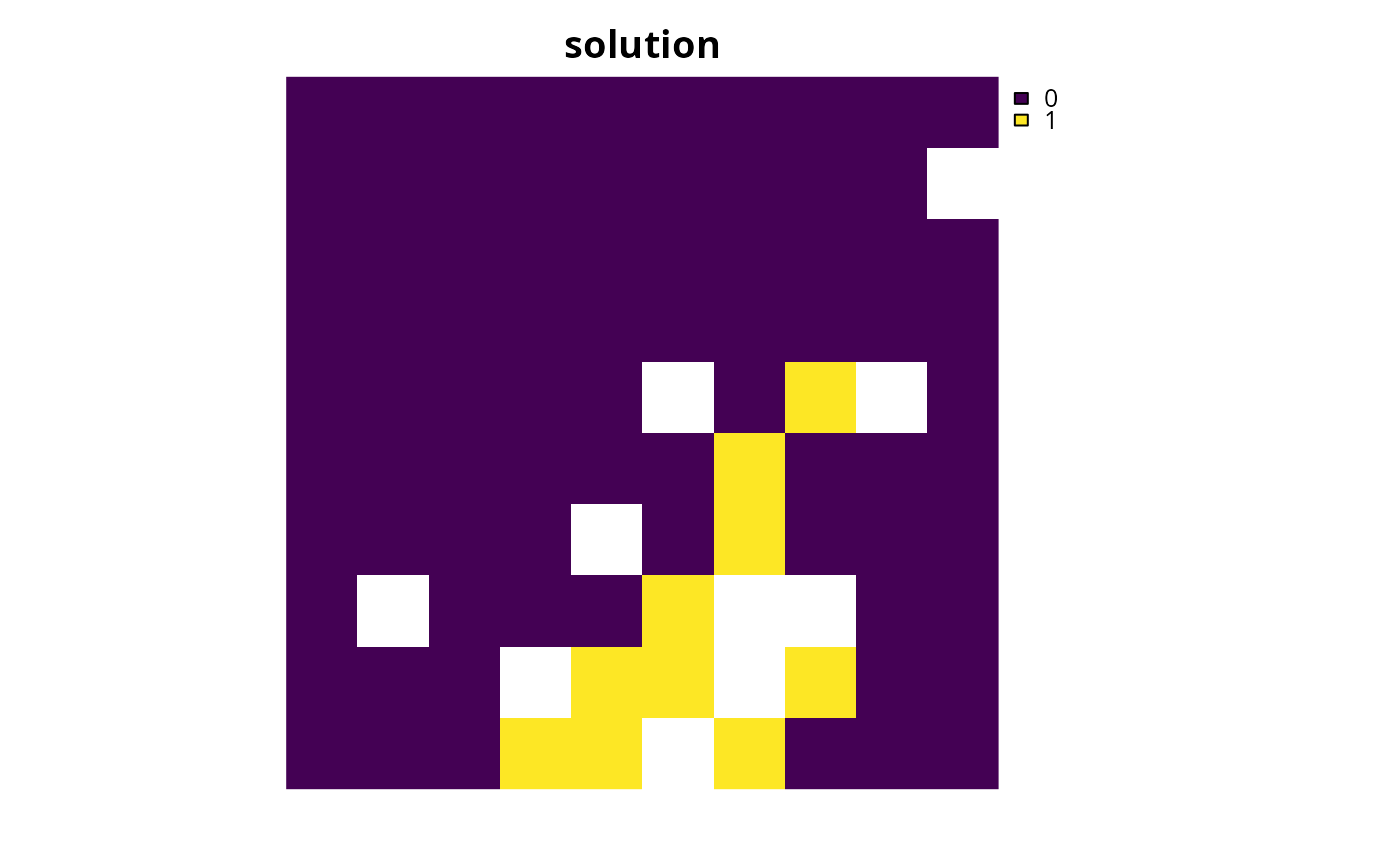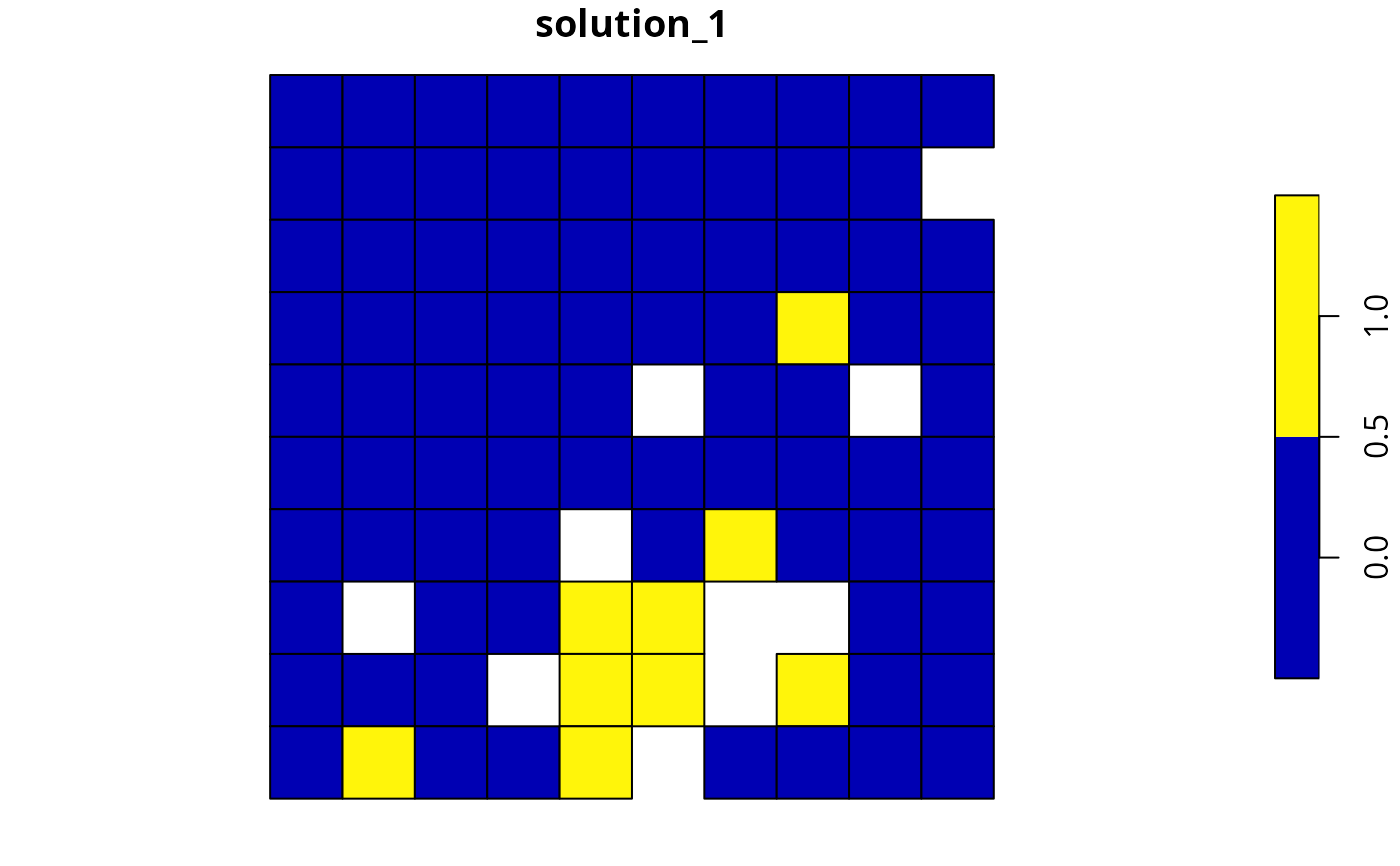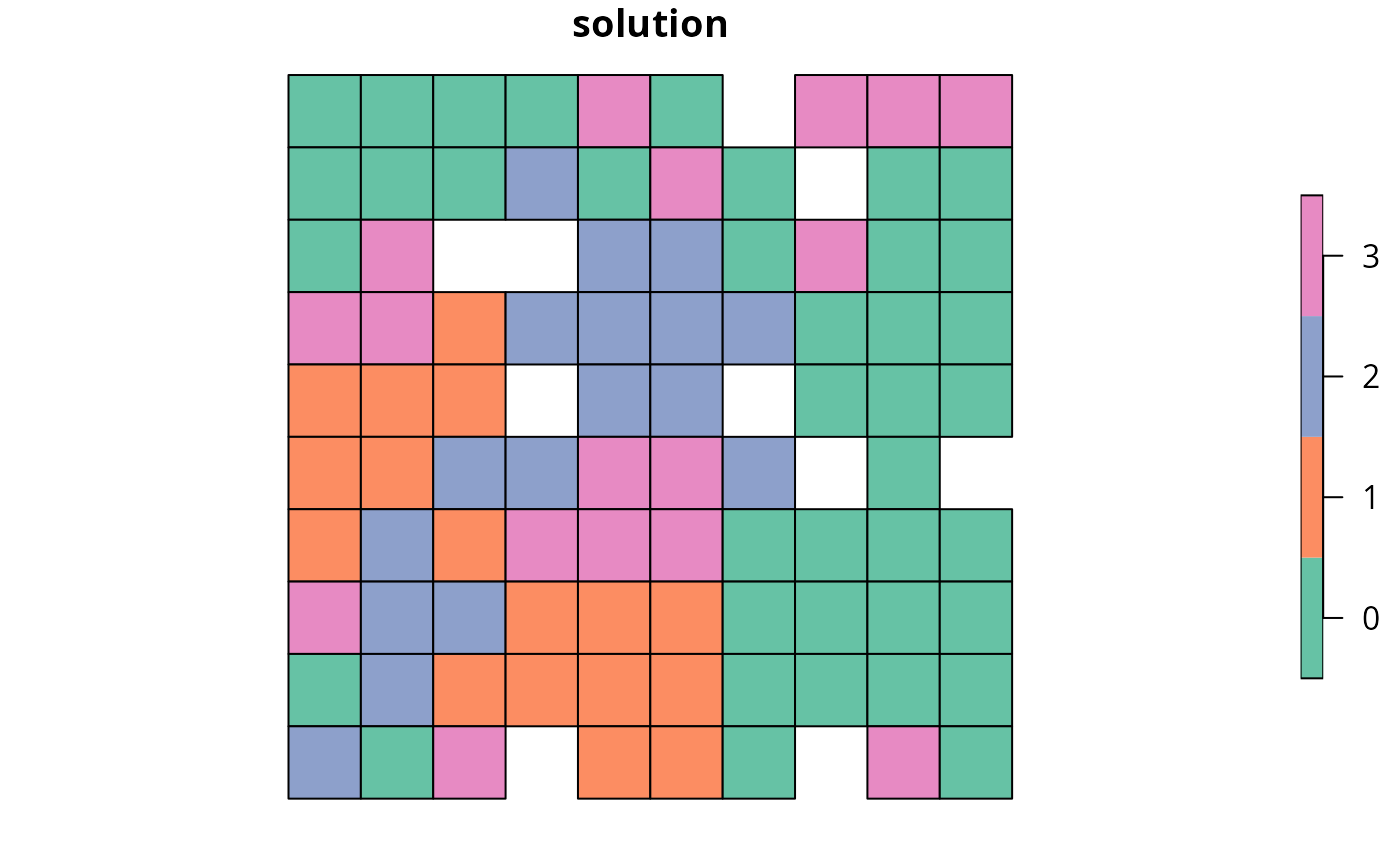Calculate the total cost of a solution to a conservation planning problem(). For example, if the planning unit cost data describe land acquisition costs (USD), then the total cost would be net cost (USD) needed to acquire all planning units selected within the solution.

eval_cost_summary(x, solution)

# S3 method for default
eval_cost_summary(x, solution)

# S3 method for ConservationProblem
eval_cost_summary(x, solution)

## Arguments

x

problem() (i.e., ConservationProblem) object.

solution

numeric, matrix, data.frame, Raster, Spatial, or sf::sf() object. The argument should be in the same format as the planning unit cost data in the argument to x. See the Solution format section for more information.

## Value

tibble::tibble() object containing the solution cost. It contains the following columns:

summary

character description of the summary statistic. The statistic associated with the "overall" value in this column is calculated using the entire solution (including all management zones if there are multiple zones). If multiple management zones are present, then summary statistics are also provided for each zone separately (indicated using zone names).

cost

numeric cost value. Greater values correspond to solutions that are more costly to implement. Thus conservation planning exercises typically prefer solutions with smaller values, because they are cheaper to implement (assuming all other relevant factors, such as feature representation, are equal).

## Details

This metric is equivalent to the Cost metric reported by the Marxan software (Ball et al. 2009). Specifically, the cost of a solution is defined as the sum of the cost values, supplied when creating a problem() object (e.g., using the cost_column argument), weighted by the status of each planning unit in the solution.

## Solution format

Broadly speaking, the argument to solution must be in the same format as the planning unit data in the argument to x. Further details on the correct format are listed separately for each of the different planning unit data formats:

x has numeric planning units

The argument to solution must be a numeric vector with each element corresponding to a different planning unit. It should have the same number of planning units as those in the argument to x. Additionally, any planning units missing cost (NA) values should also have missing (NA) values in the argument to solution.

x has matrix planning units

The argument to solution must be a matrix vector with each row corresponding to a different planning unit, and each column correspond to a different management zone. It should have the same number of planning units and zones as those in the argument to x. Additionally, any planning units missing cost (NA) values for a particular zone should also have a missing (NA) values in the argument to solution.

x has Raster planning units

The argument to solution be a Raster object where different grid cells (pixels) correspond to different planning units and layers correspond to a different management zones. It should have the same dimensionality (rows, columns, layers), resolution, extent, and coordinate reference system as the planning units in the argument to x. Additionally, any planning units missing cost (NA) values for a particular zone should also have missing (NA) values in the argument to solution.

x has data.frame planning units

The argument to solution must be a data.frame with each column corresponding to a different zone, each row corresponding to a different planning unit, and cell values corresponding to the solution value. This means that if a data.frame object containing the solution also contains additional columns, then these columns will need to be subsetted prior to using this function (see below for example with sf::sf() data). Additionally, any planning units missing cost (NA) values for a particular zone should also have missing (NA) values in the argument to solution.

x has Spatial planning units

The argument to solution must be a Spatial object with each column corresponding to a different zone, each row corresponding to a different planning unit, and cell values corresponding to the solution value. This means that if the Spatial object containing the solution also contains additional columns, then these columns will need to be subsetted prior to using this function (see below for example with sf::sf() data). Additionally, the argument to solution must also have the same coordinate reference system as the planning unit data. Furthermore, any planning units missing cost (NA) values for a particular zone should also have missing (NA) values in the argument to solution.

x has sf::sf() planning units

The argument to solution must be a sf::sf() object with each column corresponding to a different zone, each row corresponding to a different planning unit, and cell values corresponding to the solution value. This means that if the sf::sf() object containing the solution also contains additional columns, then these columns will need to be subsetted prior to using this function (see below for example). Additionally, the argument to solution must also have the same coordinate reference system as the planning unit data. Furthermore, any planning units missing cost (NA) values for a particular zone should also have missing (NA) values in the argument to solution.

See summaries for an overview of all functions for summarizing solutions.

Other summaries: eval_asym_connectivity_summary(), eval_boundary_summary(), eval_connectivity_summary(), eval_feature_representation_summary(), eval_n_summary(), eval_target_coverage_summary()

## Examples

# \dontrun{
# set seed for reproducibility
set.seed(500)

data(sim_pu_raster, sim_pu_sf, sim_features,
sim_pu_zones_sf, sim_features_zones)

# build minimal conservation problem with raster data
p1 <- problem(sim_pu_raster, sim_features) %>%

# solve the problem
s1 <- solve(p1)

# print solution
print(s1)
#> class      : RasterLayer
#> dimensions : 10, 10, 100  (nrow, ncol, ncell)
#> resolution : 0.1, 0.1  (x, y)
#> extent     : 0, 1, 0, 1  (xmin, xmax, ymin, ymax)
#> crs        : NA
#> source     : memory
#> names      : layer
#> values     : 0, 1  (min, max)
#>

# plot solution
plot(s1, main = "solution", axes = FALSE, box = FALSE)# calculate cost of the solution
r1 <- eval_cost_summary(p1, s1)
print(r1)
#> # A tibble: 1 × 2
#>   summary  cost
#>   <chr>   <dbl>
#> 1 overall 1987.

# build minimal conservation problem with polygon (sf) data
p2 <- problem(sim_pu_sf, sim_features, cost_column = "cost") %>%

# solve the problem
s2 <- solve(p2)

# plot solution
plot(s2[, "solution_1"])# print first six rows of the attribute table
#> Simple feature collection with 6 features and 4 fields
#> Geometry type: POLYGON
#> Dimension:     XY
#> Bounding box:  xmin: 0 ymin: 0.9 xmax: 0.6 ymax: 1
#> CRS:           NA
#>       cost locked_in locked_out solution_1                       geometry
#> 1 215.8638     FALSE      FALSE          0 POLYGON ((0 1, 0.1 1, 0.1 0...
#> 2 212.7823     FALSE      FALSE          0 POLYGON ((0.1 1, 0.2 1, 0.2...
#> 3 207.4962     FALSE      FALSE          0 POLYGON ((0.2 1, 0.3 1, 0.3...
#> 4 208.9322     FALSE       TRUE          0 POLYGON ((0.3 1, 0.4 1, 0.4...
#> 5 214.0419     FALSE      FALSE          0 POLYGON ((0.4 1, 0.5 1, 0.5...
#> 6 213.7636     FALSE      FALSE          0 POLYGON ((0.5 1, 0.6 1, 0.6...

# calculate cost of the solution
r2 <- eval_cost_summary(p2, s2[, "solution_1"])
print(r2)
#> # A tibble: 1 × 2
#>   summary  cost
#>   <chr>   <dbl>
#> 1 overall 1793.

# manually calculate cost of the solution
r2_manual <- sum(s2$solution * sim_pu_sf$cost, na.rm = TRUE)
print(r2_manual)
#>  1792.535

# build multi-zone conservation problem with polygon (sf) data
p3 <- problem(sim_pu_zones_sf, sim_features_zones,
cost_column = c("cost_1", "cost_2", "cost_3")) %>%
add_relative_targets(matrix(runif(15, 0.1, 0.2), nrow = 5,
ncol = 3)) %>%

# solve the problem
s3 <- solve(p3)

# print first six rows of the attribute table
#> Simple feature collection with 6 features and 9 fields
#> Geometry type: POLYGON
#> Dimension:     XY
#> Bounding box:  xmin: 0 ymin: 0.9 xmax: 0.6 ymax: 1
#> CRS:           NA
#>     cost_1   cost_2   cost_3 locked_1 locked_2 locked_3 solution_1_zone_1
#> 1 215.8638 183.3344 205.4113    FALSE    FALSE    FALSE                 0
#> 2 212.7823 189.4978 209.6404    FALSE    FALSE    FALSE                 0
#> 3 207.4962 193.6007 215.4212     TRUE    FALSE    FALSE                 0
#> 4 208.9322 197.5897 218.5241    FALSE    FALSE    FALSE                 0
#> 5 214.0419 199.8033 220.7100    FALSE    FALSE    FALSE                 0
#> 6 213.7636 203.1867 224.6809    FALSE    FALSE    FALSE                 0
#>   solution_1_zone_2 solution_1_zone_3                       geometry
#> 1                 0                 0 POLYGON ((0 1, 0.1 1, 0.1 0...
#> 2                 0                 1 POLYGON ((0.1 1, 0.2 1, 0.2...
#> 3                 0                 0 POLYGON ((0.2 1, 0.3 1, 0.3...
#> 4                 0                 1 POLYGON ((0.3 1, 0.4 1, 0.4...
#> 5                 0                 1 POLYGON ((0.4 1, 0.5 1, 0.5...
#> 6                 0                 0 POLYGON ((0.5 1, 0.6 1, 0.6...

# create new column representing the zone id that each planning unit
# was allocated to in the solution
s3$solution <- category_vector( s3[, c("solution_1_zone_1", "solution_1_zone_2", "solution_1_zone_3")]) s3$solution <- factor(s3\$solution)

# plot solution
plot(s3[, "solution"])# calculate cost of the solution
r3 <- eval_cost_summary(
p3, s3[, c("solution_1_zone_1", "solution_1_zone_2", "solution_1_zone_3")])
print(r3)
#> # A tibble: 4 × 2
#>   summary   cost
#>   <chr>    <dbl>
#> 1 overall 10559.
#> 2 zone_1   3409.
#> 3 zone_2   3513.
#> 4 zone_3   3638.
# }